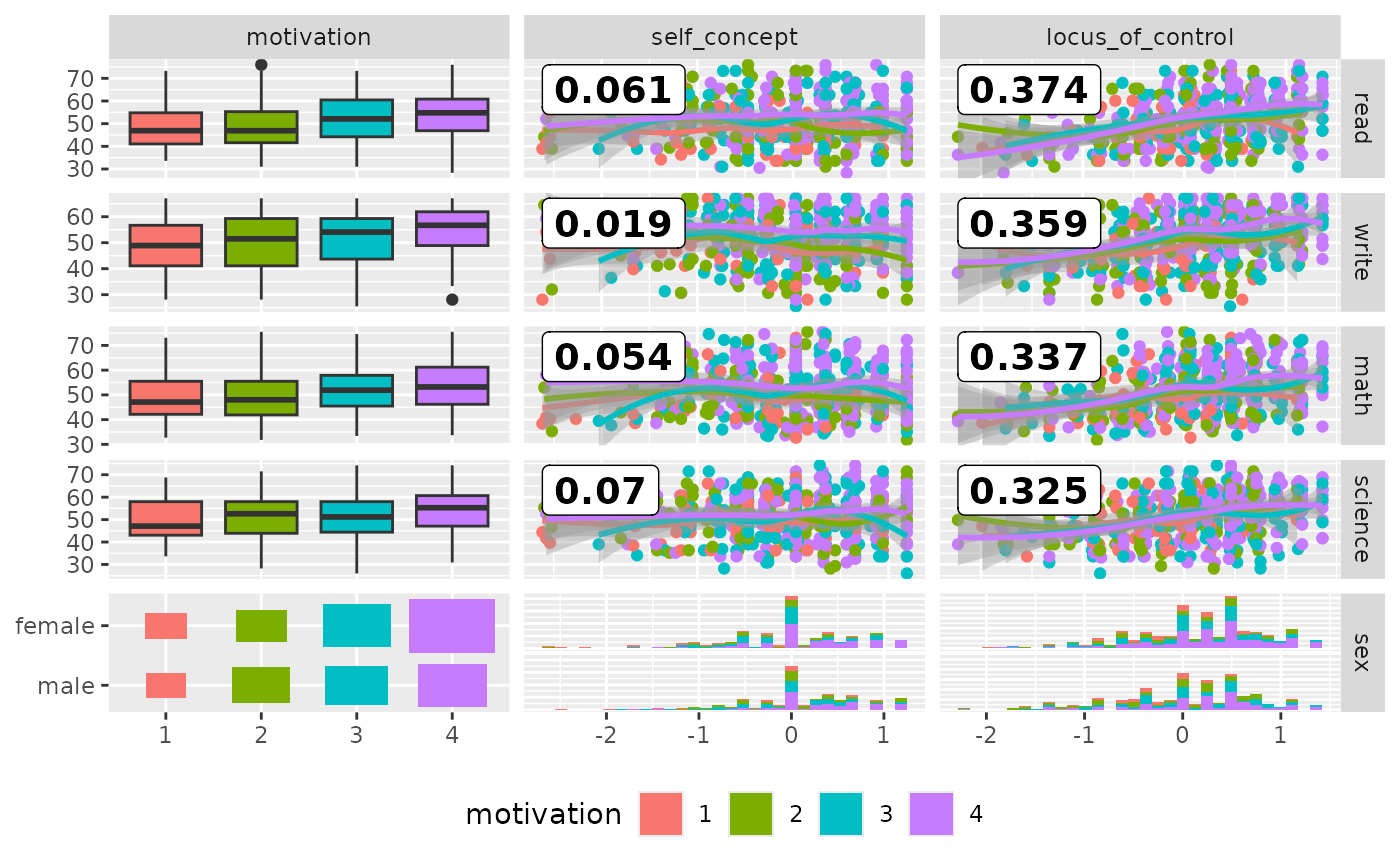Make a matrix of plots with a given data set with two different column sets

ggduo(
data,
mapping = NULL,
columnsX = 1:ncol(data),
columnsY = 1:ncol(data),
title = NULL,
types = list(continuous = "smooth_loess", comboVertical = "box_no_facet",
comboHorizontal = "facethist", discrete = "count"),
axisLabels = c("show", "none"),
columnLabelsX = colnames(data[columnsX]),
columnLabelsY = colnames(data[columnsY]),
labeller = "label_value",
switch = NULL,
xlab = NULL,
ylab = NULL,
showStrips = NULL,
legend = NULL,
cardinality_threshold = 15,
progress = NULL,
xProportions = NULL,
yProportions = NULL,
legends = stop("deprecated")
)

## Arguments

data data set using. Can have both numerical and categorical data. aesthetic mapping (besides x and y). See aes(). If mapping is numeric, columns will be set to the mapping value and mapping will be set to NULL. which columns are used to make plots. Defaults to all columns. title, x label, and y label for the graph see Details either "show" to display axisLabels or "none" for no axis labels label names to be displayed. Defaults to names of columns being used. labeller for facets. See labellers. Common values are "label_value" (default) and "label_parsed". switch parameter for facet_grid. See ggplot2::facet_grid. By default, the labels are displayed on the top and right of the plot. If "x", the top labels will be displayed to the bottom. If "y", the right-hand side labels will be displayed to the left. Can also be set to "both" boolean to determine if each plot's strips should be displayed. NULL will default to the top and right side plots only. TRUE or FALSE will turn all strips on or off respectively. May be the two objects described below or the default NULL value. The legend position can be moved by using ggplot2's theme element pm + theme(legend.position = "bottom") a single numeric valueprovides the location of a plot according to the display order. Such as legend = 3 in a plot matrix with 2 rows and 5 columns displayed by column will return the plot in position c(1,2)a object from grab_legend()a predetermined plot legend that will be displayed directly maximum number of levels allowed in a character / factor column. Set this value to NULL to not check factor columns. Defaults to 15 NULL (default) for a progress bar in interactive sessions with more than 15 plots, TRUE for a progress bar, FALSE for no progress bar, or a function that accepts at least a plot matrix and returns a new progress::progress_bar. See ggmatrix_progress. Value to change how much area is given for each plot. Either NULL (default), numeric value matching respective length, grid::unit object with matching respective length or "auto" for automatic relative proportions based on the number of levels for categorical variables. deprecated

## Details

types is a list that may contain the variables 'continuous', 'combo', 'discrete', and 'na'. Each element of the list may be a function or a string. If a string is supplied, If a string is supplied, it must be a character string representing the tail end of a ggally_NAME function. The list of current valid ggally_NAME functions is visible in a dedicated vignette.

continuous

This option is used for continuous X and Y data.

comboHorizontal

This option is used for either continuous X and categorical Y data or categorical X and continuous Y data.

comboVertical

This option is used for either continuous X and categorical Y data or categorical X and continuous Y data.

discrete

This option is used for categorical X and Y data.

na

This option is used when all X data is NA, all Y data is NA, or either all X or Y data is NA.

If 'blank' is ever chosen as an option, then ggduo will produce an empty plot.

If a function is supplied as an option, it should implement the function api of function(data, mapping, ...){#make ggplot2 plot}. If a specific function needs its parameters set, wrap(fn, param1 = val1, param2 = val2) the function with its parameters.

## Examples

 # small function to display plots only if it's interactive
p_ <- GGally::print_if_interactive

data(baseball, package = "plyr")

# Keep players from 1990-1995 with at least one at bat
# Add how many singles a player hit
# (must do in two steps as X1b is used in calculations)
dt <- transform(
subset(baseball, year >= 1990 & year <= 1995 & ab > 0),
X1b = h - X2b - X3b - hr
)
#  the player's batting average,
#  the player's slugging percentage,
#  and the player's on base percentage
# Make factor a year, as each season is discrete
dt <- transform(
dt,
batting_avg = h / ab,
slug = (X1b + 2*X2b + 3*X3b + 4*hr) / ab,
on_base = (h + bb + hbp) / (ab + bb + hbp),
year = as.factor(year)
)

pm <- ggduo(
dt,
c("year", "g", "ab", "lg"),
c("batting_avg", "slug", "on_base"),
mapping = ggplot2::aes(color = lg)
)
# Prints, but
#   there is severe over plotting in the continuous plots
#   the labels could be better
#   want to add more hitting information
p_(pm)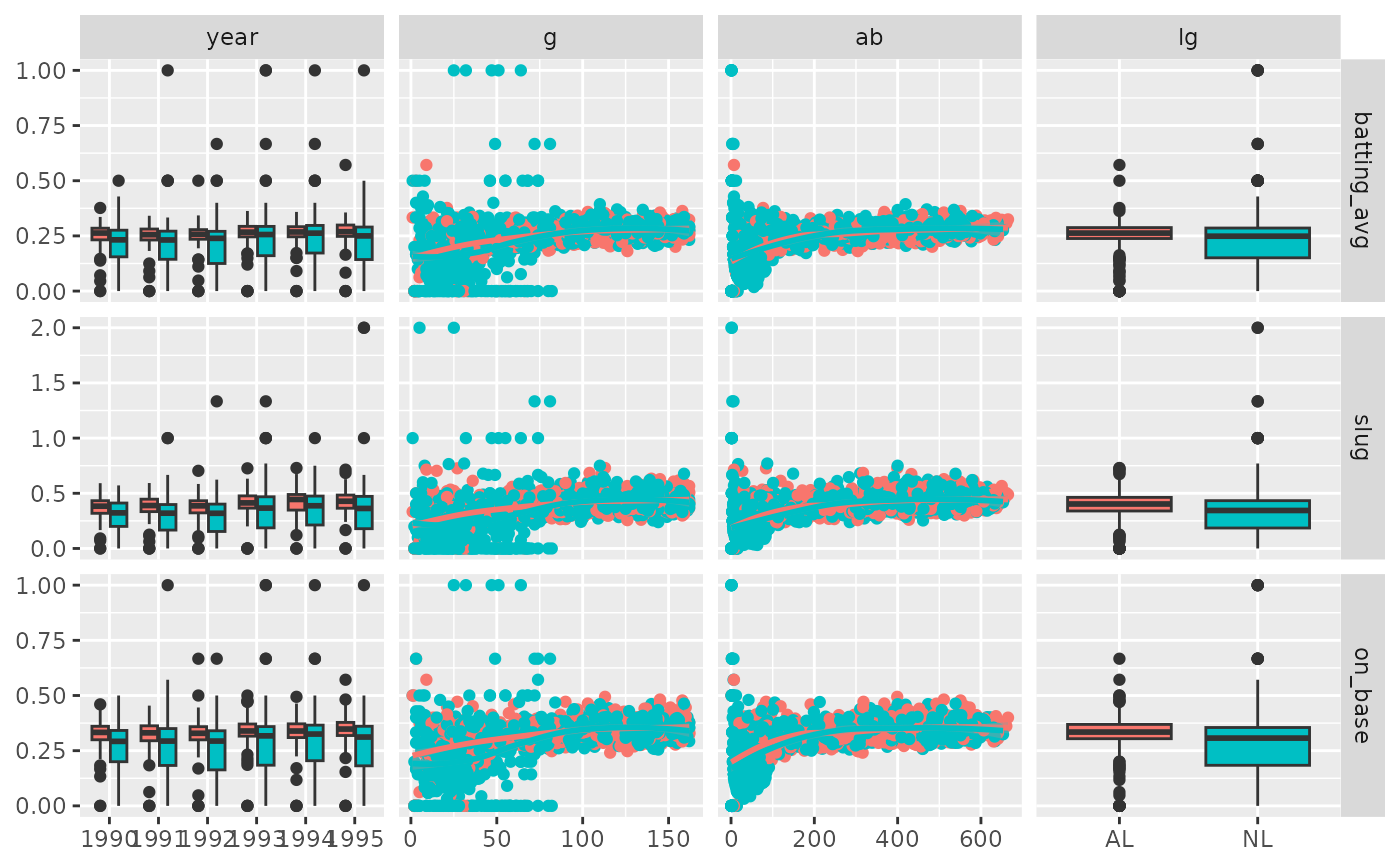pm <- ggduo(
dt,
c("year", "g", "ab", "lg"),
c("batting_avg", "slug", "on_base"),
columnLabelsX = c("year", "player game count", "player at bat count", "league"),
columnLabelsY = c("batting avg", "slug %", "on base %"),
title = "Baseball Hitting Stats from 1990-1995",
mapping = ggplot2::aes(color = lg),
types = list(
# change the shape and add some transparency to the points
continuous = wrap("smooth_loess", alpha = 0.50, shape = "+")
),
showStrips = FALSE
)

p_(pm)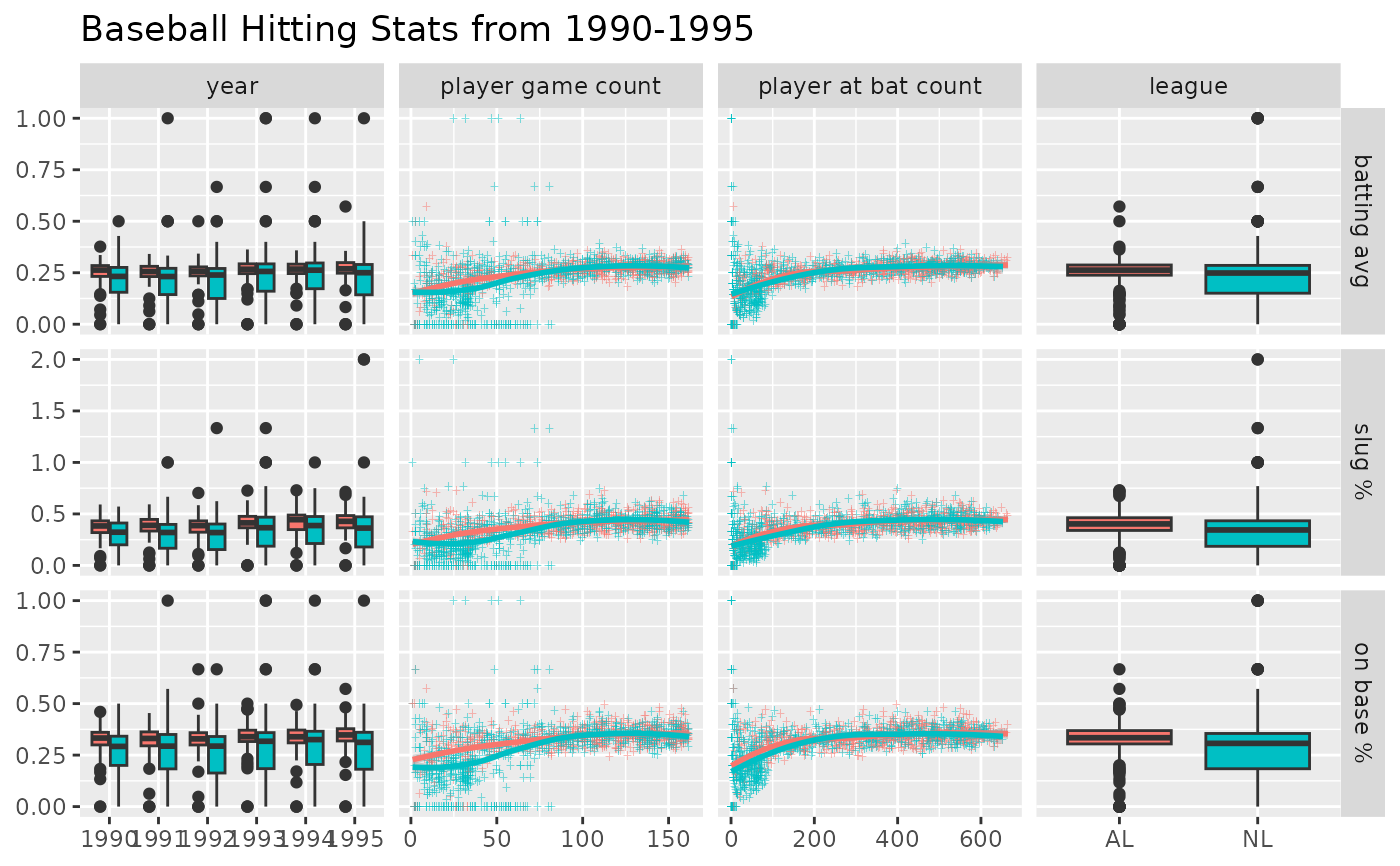# Use "auto" to adapt width of the sub-plots
pm <- ggduo(
dt,
c("year", "g", "ab", "lg"),
c("batting_avg", "slug", "on_base"),
mapping = ggplot2::aes(color = lg),
xProportions = "auto"
)

p_(pm)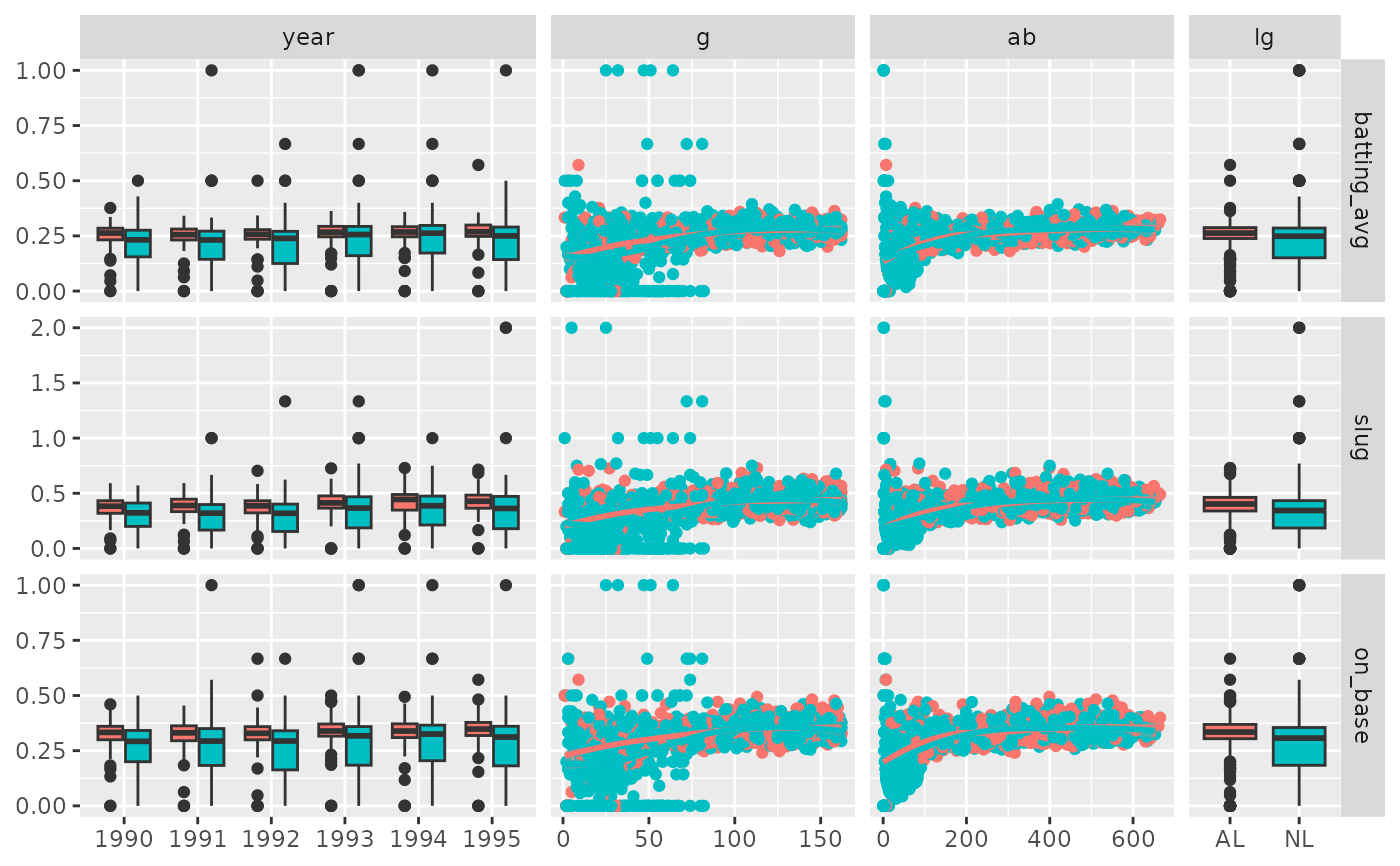# Custom widths & heights of the sub-plots
pm <- ggduo(
dt,
c("year", "g", "ab", "lg"),
c("batting_avg", "slug", "on_base"),
mapping = ggplot2::aes(color = lg),
xProportions = c(6, 4, 3, 2),
yProportions = c(1, 2, 1)
)

p_(pm)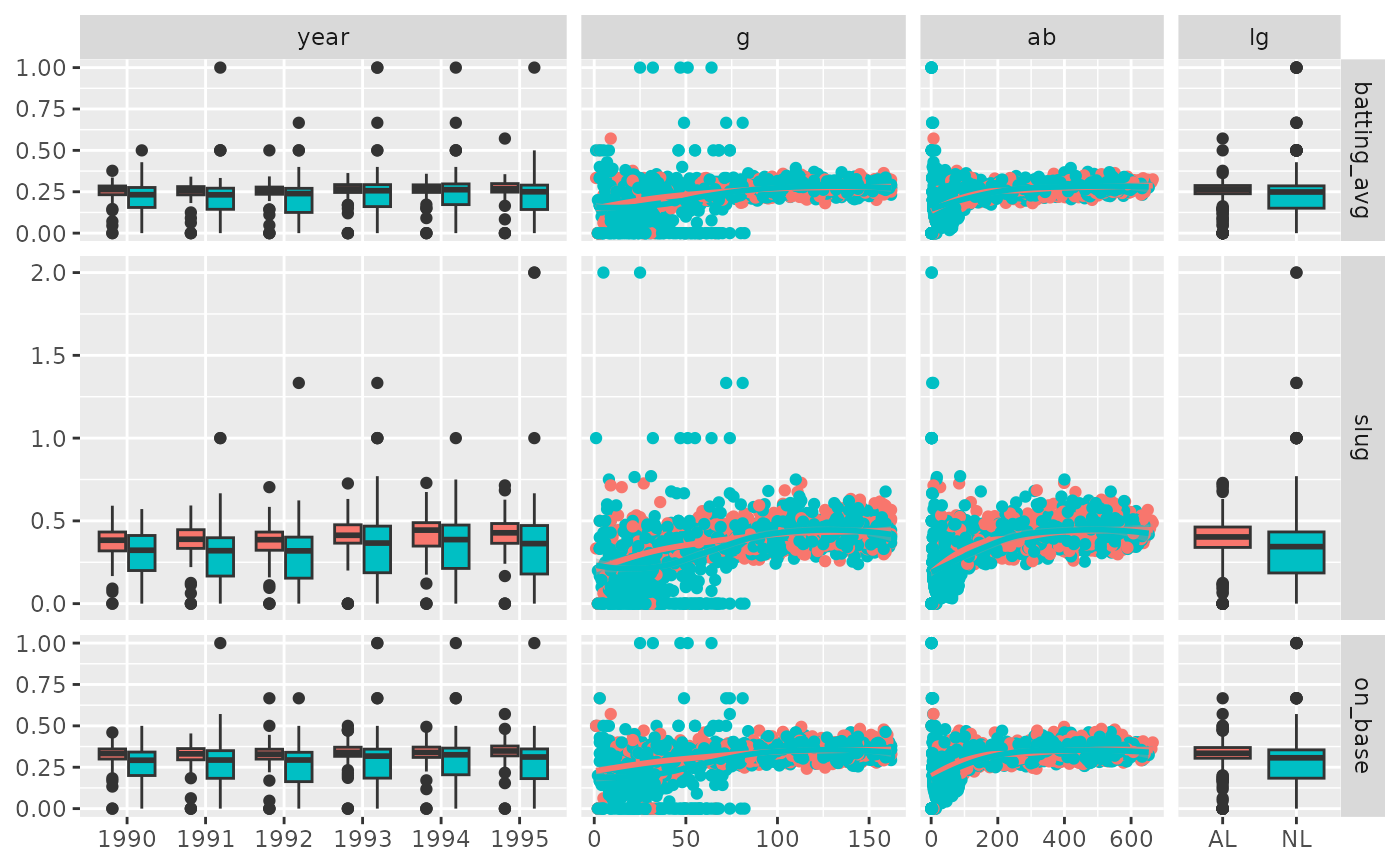# Example derived from:
## R Data Analysis Examples | Canonical Correlation Analysis.  UCLA: Institute for Digital
##   Research and Education.
##   from http://www.stats.idre.ucla.edu/r/dae/canonical-correlation-analysis
##   (accessed May 22, 2017).
# "Example 1. A researcher has collected data on three psychological variables, four
#  academic variables (standardized test scores) and gender for 600 college freshman.
#  She is interested in how the set of psychological variables relates to the academic
#  variables and gender. In particular, the researcher is interested in how many
#  dimensions (canonical variables) are necessary to understand the association between
#  the two sets of variables."
#>  Min.   :-2.23000   Min.   :-2.620000   Length:600         Min.   :28.3
#>  1st Qu.:-0.37250   1st Qu.:-0.300000   Class :character   1st Qu.:44.2
#>  Median : 0.21000   Median : 0.030000   Mode  :character   Median :52.1
#>  Mean   : 0.09653   Mean   : 0.004917                      Mean   :51.9
#>  3rd Qu.: 0.51000   3rd Qu.: 0.440000                      3rd Qu.:60.1
#>  Max.   : 1.36000   Max.   : 1.190000                      Max.   :76.0
#>      write            math          science          sex
#>  Min.   :25.50   Min.   :31.80   Min.   :26.00   Length:600
#>  1st Qu.:44.30   1st Qu.:44.50   1st Qu.:44.40   Class :character
#>  Median :54.10   Median :51.30   Median :52.60   Mode  :character
#>  Mean   :52.38   Mean   :51.85   Mean   :51.76
#>  3rd Qu.:59.90   3rd Qu.:58.38   3rd Qu.:58.65
#>  Max.   :67.10   Max.   :75.50   Max.   :74.20
#>  "read"    "write"   "math"    "science" "sex"
## Within correlation
#> stat_bin() using bins = 30. Pick better value with binwidth.#> stat_bin() using bins = 30. Pick better value with binwidth.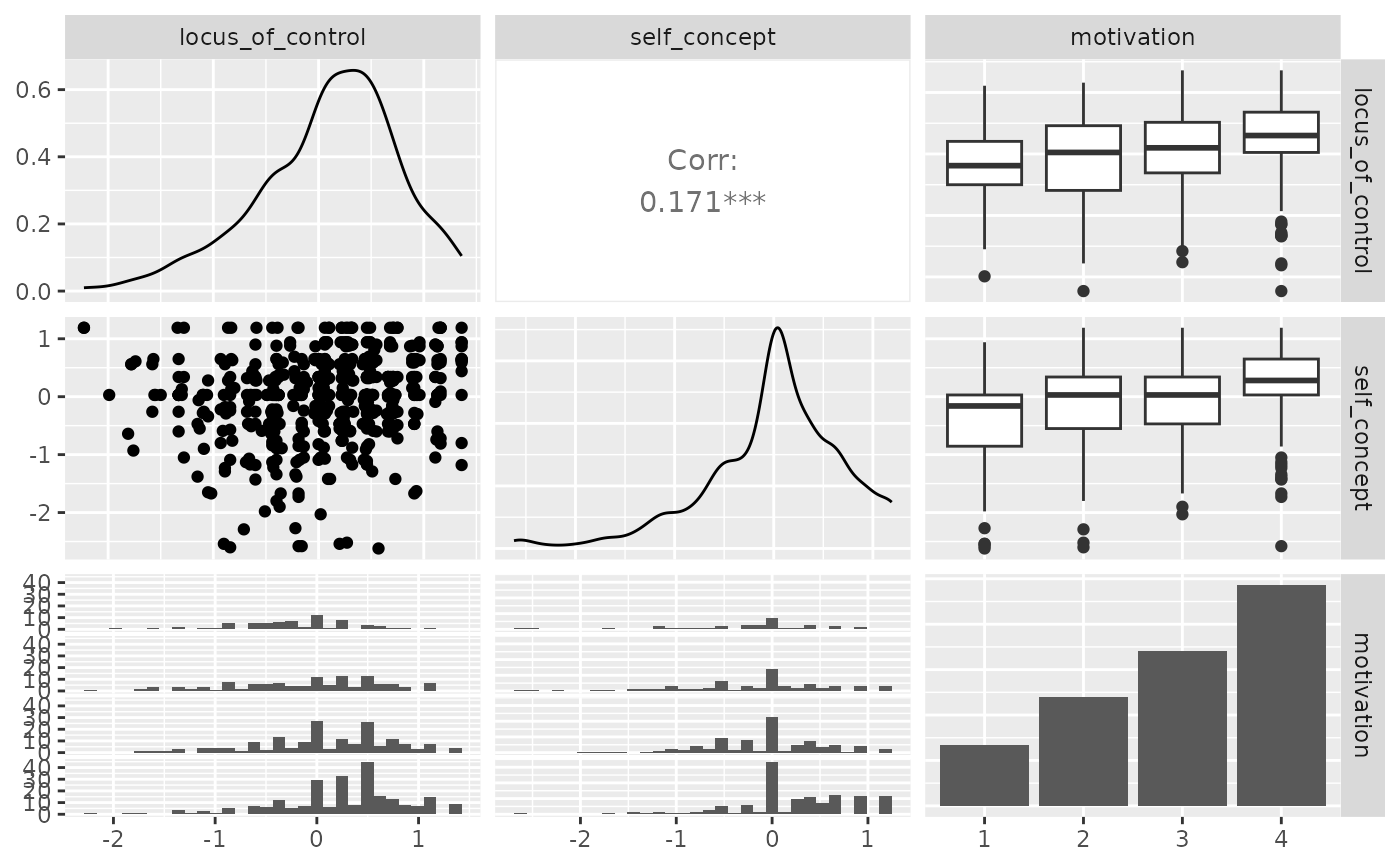p_(ggpairs(psychademic, columns = academic_variables))
#> stat_bin() using bins = 30. Pick better value with binwidth.#> stat_bin() using bins = 30. Pick better value with binwidth.#> stat_bin() using bins = 30. Pick better value with binwidth.#> stat_bin() using bins = 30. Pick better value with binwidth.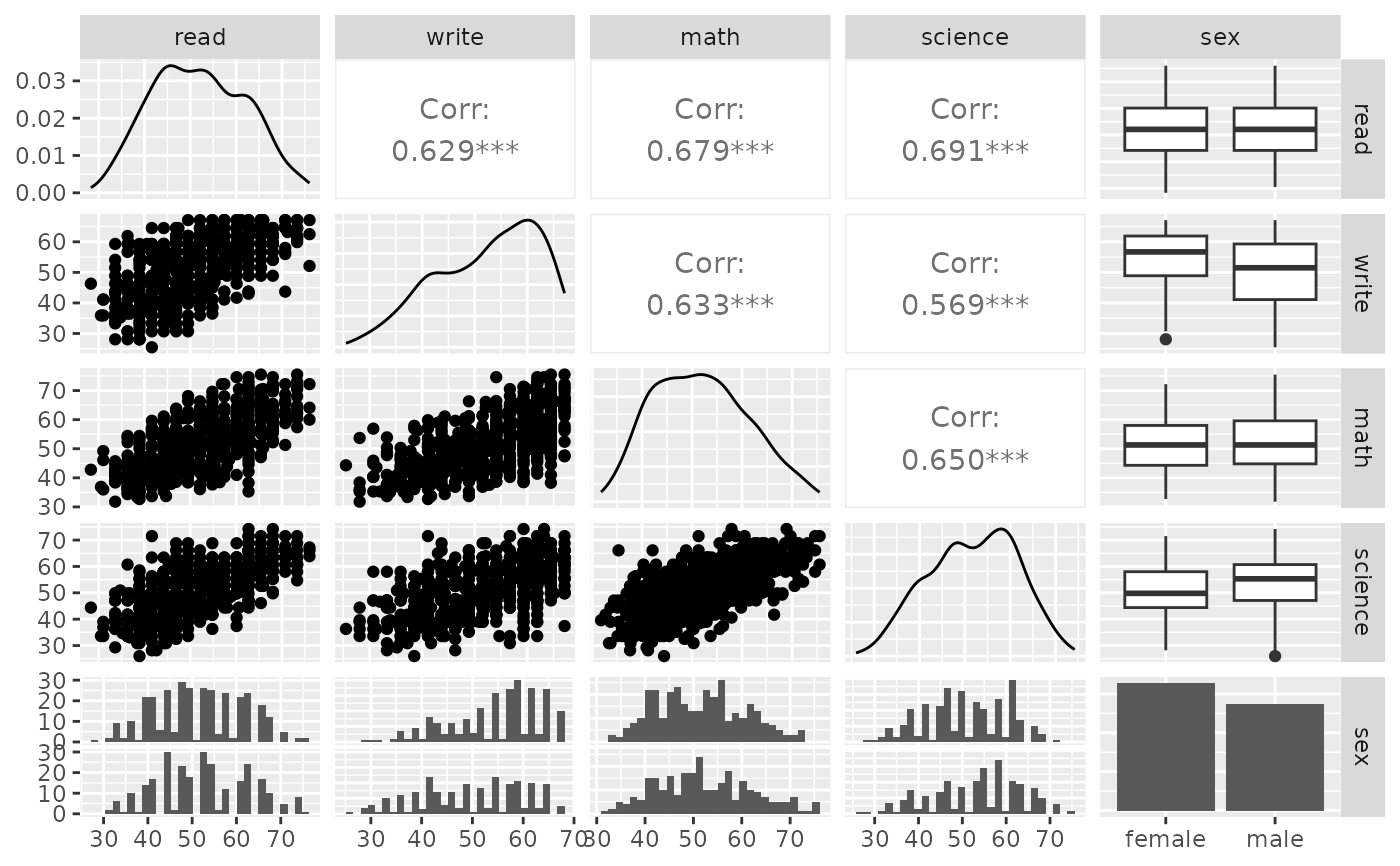## Between correlation
loess_with_cor <- function(data, mapping, ..., method = "pearson") {
x <- eval_data_col(data, mapping$x) y <- eval_data_col(data, mapping$y)
cor <- cor(x, y, method = method)
ggally_smooth_loess(data, mapping, ...) +
ggplot2::geom_label(
data = data.frame(
x = min(x, na.rm = TRUE),
y = max(y, na.rm = TRUE),
lab = round(cor, digits = 3)
),
mapping = ggplot2::aes(x = x, y = y, label = lab),
hjust = 0, vjust = 1,
size = 5, fontface = "bold",
inherit.aes = FALSE # do not inherit anything from the ...
)
}
pm <- ggduo(
types = list(continuous = loess_with_cor),
showStrips = FALSE
)
suppressWarnings(p_(pm)) # ignore warnings from loess
#> stat_bin() using bins = 30. Pick better value with binwidth.#> stat_bin() using bins = 30. Pick better value with binwidth.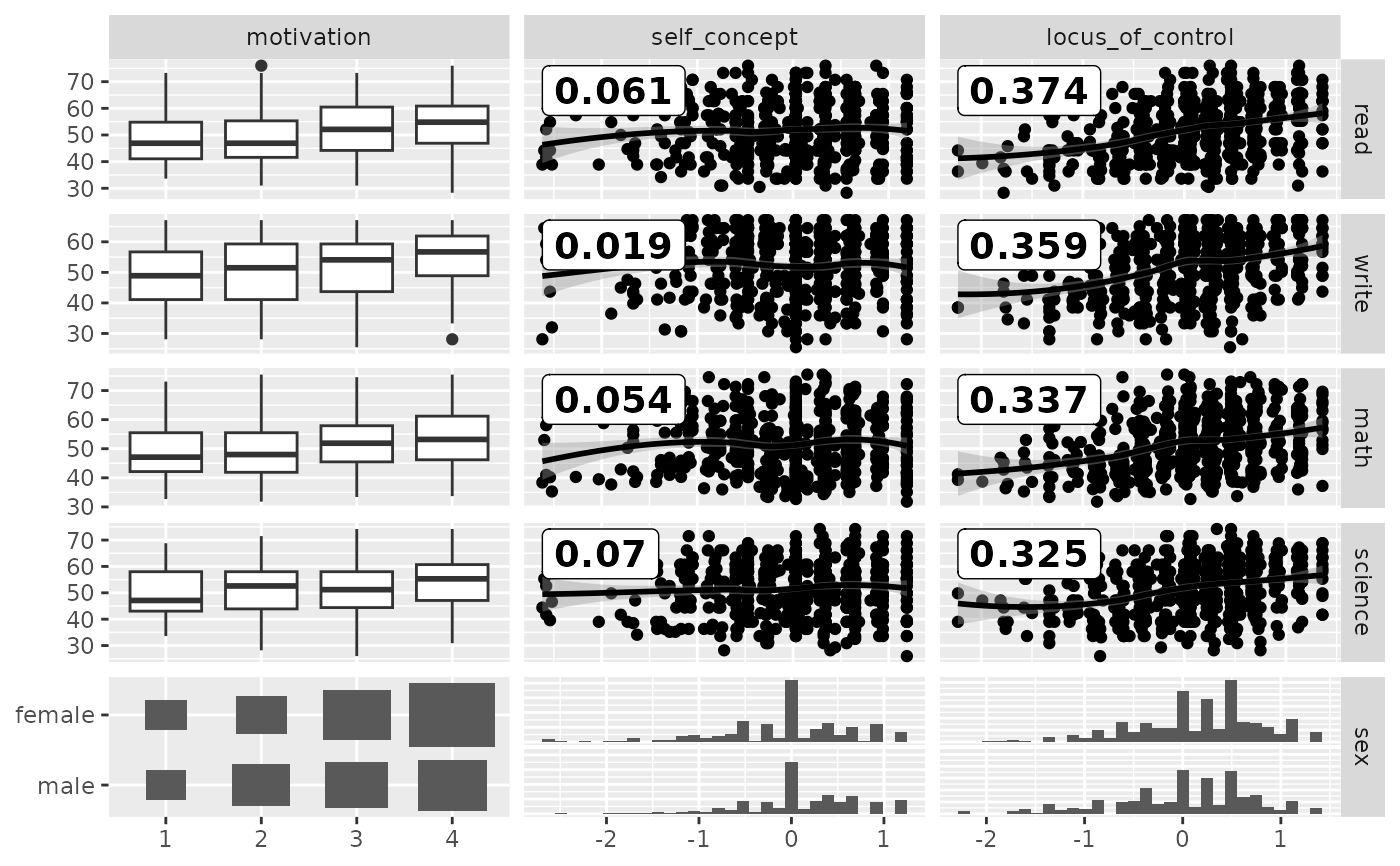# add color according to sex
pm <- ggduo(
mapping = ggplot2::aes(color = sex),
types = list(continuous = loess_with_cor),
showStrips = FALSE,
legend = c(5,2)
)
suppressWarnings(p_(pm))
#> stat_bin() using bins = 30. Pick better value with binwidth.#> stat_bin() using bins = 30. Pick better value with binwidth.#> stat_bin() using bins = 30. Pick better value with binwidth.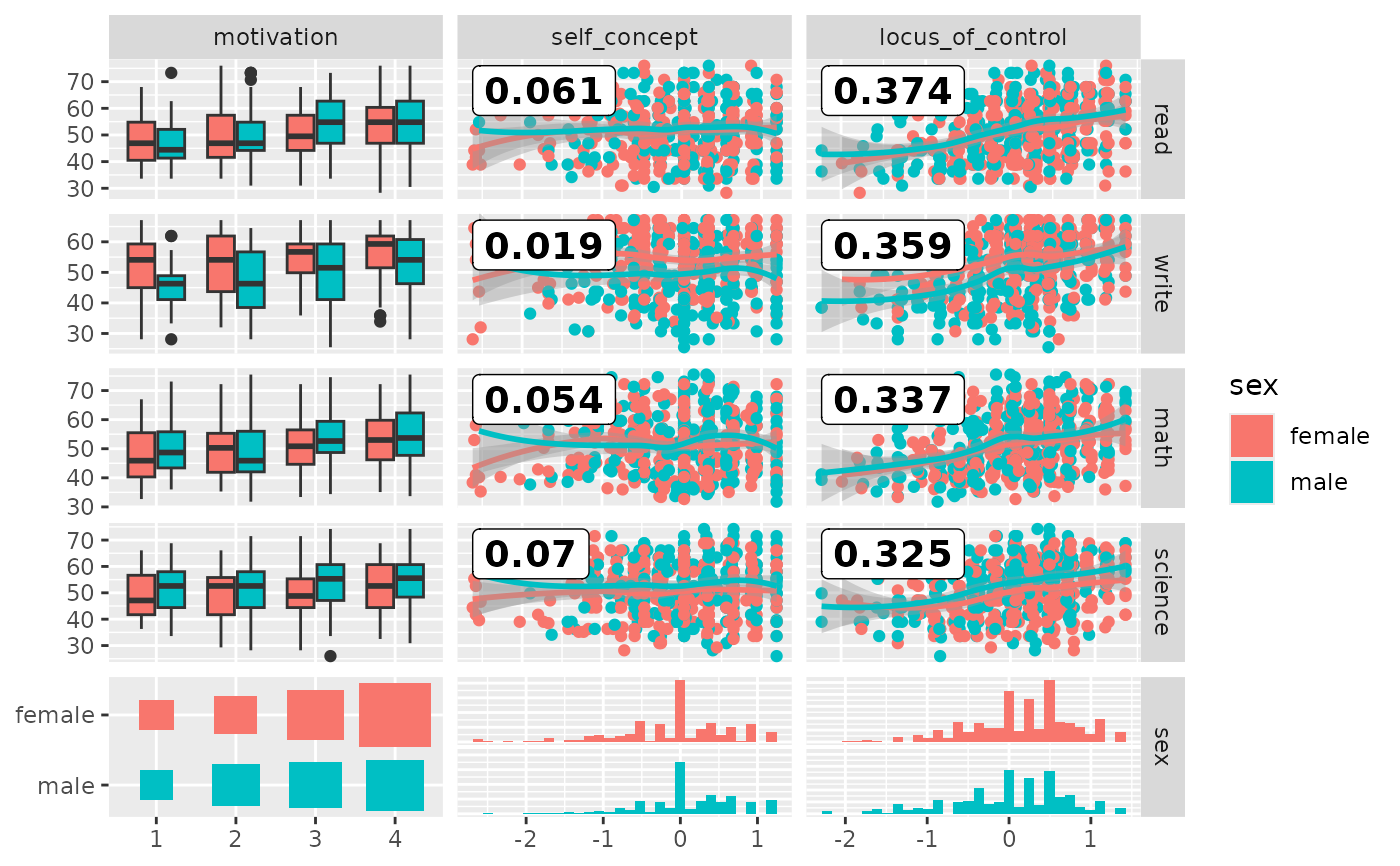# add color according to sex
pm <- ggduo(
#> stat_bin() using bins = 30. Pick better value with binwidth.#> stat_bin() using bins = 30. Pick better value with binwidth.#> stat_bin() using bins = 30. Pick better value with binwidth.